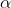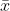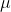##### Question

In: Statistics and Probability

# Calculate the margin of error and construct the confidence interval for the population mean using the...

Calculate the margin of error and construct the confidence interval for the population mean using the Student's t-distribution (you may assume the population data is normally distributed).

T-Distribution Table

a.  x̄ =87.3, n=64, s=19.6, x̄ =87.3, n=64, s=19.6, 98% confidence

E=E=

Round to two decimal places

<  μ  <  <  μ  <

Round to two decimal places

b.  x̄ =31.6, n=44, s=14.6, x̄ =31.6, n=44, s=14.6, 90% confidence

E=E=

Round to two decimal places

<  μ  <  <  μ  <

Round to two decimal places

## Solutions

##### Expert Solution

Solution :

a) degrees of freedom = n - 1 = 64 - 1 = 63

t/2,df = t0.01,63 = 2.387

Margin of error = E = t/2,df * (s /n)

= 2.387 * ( 19.6 / 64)

Margin of error = E = 5.85

The 98% confidence interval estimate of the population mean is,- E <<+ E

87.3 - 5.85 << 87.3 + 5.85

( 81.45 << 93.15 )

b) degrees of freedom = n - 1 = 44 - 1 = 43

t/2,df = t0.05,43 = 1.681

Margin of error = E = t/2,df * (s /n)

= 1.681 * ( 14.6 / 44)

Margin of error = E = 3.70

The 90% confidence interval estimate of the population mean is,- E <<+ E

31.6 - 3.70 << 31.6 + 3.70

( 27.90 << 35.30 )

## Related Solutions

##### Calculate the margin of error and construct a confidence interval for the population proportion using the...
Calculate the margin of error and construct a confidence interval for the population proportion using the normal approximation to the  p̂  p̂ -distribution (if it is appropriate to do so). Standard Normal Distribution Table a.  p̂ =0.9, n=160,  α =0.2 p̂ =0.9, n=160,  α =0.2 E=E= Round to four decimal places Enter 0 if normal approximation cannot be used   < p <  < p <   Round to four decimal places Enter 0 if normal approximation cannot be used b.  p̂ =0.45, n=140,  α =0.2 p̂ =0.45, n=140,  α =0.2...
##### Match the margin of error for an 80% confidence interval to estimate the population mean with...
Match the margin of error for an 80% confidence interval to estimate the population mean with sigma σ equals=50 with its corresponding sample sizes. Question 5 options: a.) 8.07. 1.) n= 34 b.) 9.45 2.) n= 46 c.) 10.99 3.) n= 63
##### Determine the margin of error for a confidence interval to estimate the population mean with nequals24...
Determine the margin of error for a confidence interval to estimate the population mean with nequals24 and s​ = 13.5 for the confidence levels below. ​a) 80​% ​b) 90​% ​c) 99​%
##### Determine the margin of error for a confidence interval to estimate the population mean with n=34...
Determine the margin of error for a confidence interval to estimate the population mean with n=34 and σ=48 for the following confidence levels. 91% 95% 98%
##### Determine the margin of error for a confidence interval to estimate the population mean with n=22...
Determine the margin of error for a confidence interval to estimate the population mean with n=22 and s​ =14.4 for the confidence levels below a. 80% b.90% c. 99% The margin of error for an 80​% confidence interval is_______? repeat for 90% and 99%
##### A confidence interval for a population mean has length 26. a) Determine the margin of error....
A confidence interval for a population mean has length 26. a) Determine the margin of error. b) If the sample mean is 58.9, obtain the confidence interval. Confidence interval: ( , ). Lindsey thinks a certain potato chip maker is putting fewer chips in their regular bags of chips. From a random sample of 23 bags of potato chips she calculated a P value of 0.029 for the sample. (a) At a 5% level of significance, is there evidence that...
##### Determine the margin of error for a 99​% confidence interval to estimate the population mean when...
Determine the margin of error for a 99​% confidence interval to estimate the population mean when s​ = 43 for the sample sizes below. ​a) n=12 ​b) n=25 ​c) n=46 ​a) The margin of error for a 99​% confidence interval when n=12 is _.
##### Determine the margin of error for a 99% confidence interval to estimate the population mean when...
Determine the margin of error for a 99% confidence interval to estimate the population mean when s=45 for the sample sizes of n=15, n=34, n=54. (Find the margin of error for each interval when n=x) Determine the margin of error for a confidence interval to estimate the population mean with n=24 and s=12.3 for confidence levels 80%, 90%, 99%.
##### Determine the margin of error for a confidence interval to estimate the population mean with n=18...
Determine the margin of error for a confidence interval to estimate the population mean with n=18 and s=12.1 for the confidence levels below. A) The margin of error for an 80% confidence interval is: B) The margin of error for an 90% confidence interval is: C) The margin of error for an 99% confidence interval is:
##### Determine the margin of error for a confidence interval to estimate the population proportion for the...
Determine the margin of error for a confidence interval to estimate the population proportion for the following confidence levels with a sample proportion equal to 0.36 and n=125. a. 90​%             b. 95​%             c. 98​% a. The margin of error for a confidence interval to estimate the population proportion for the 90% confidence level is _ b. The margin of error for a confidence interval to estimate the population proportion for the 95% confidence level is _ c. The margin of...